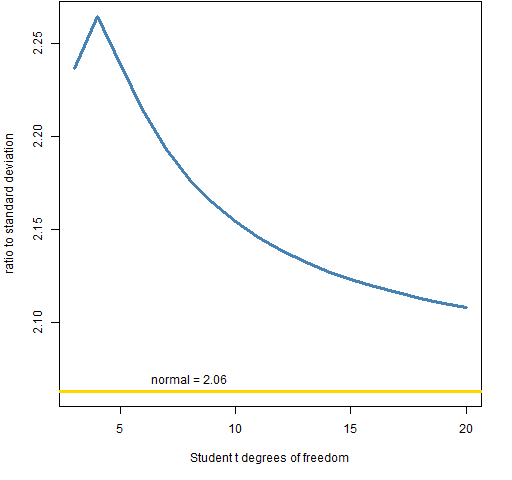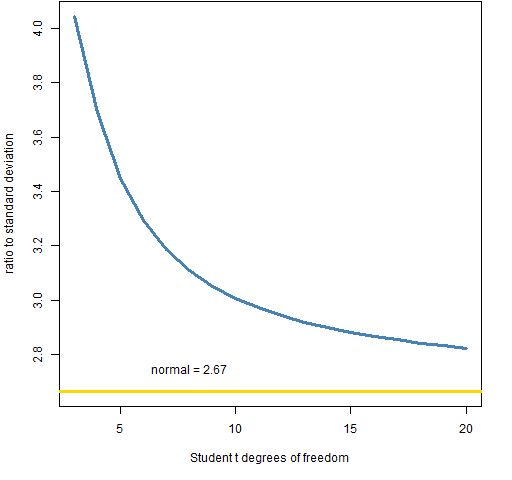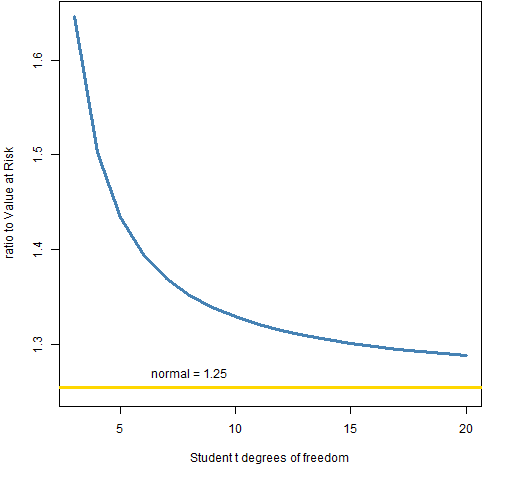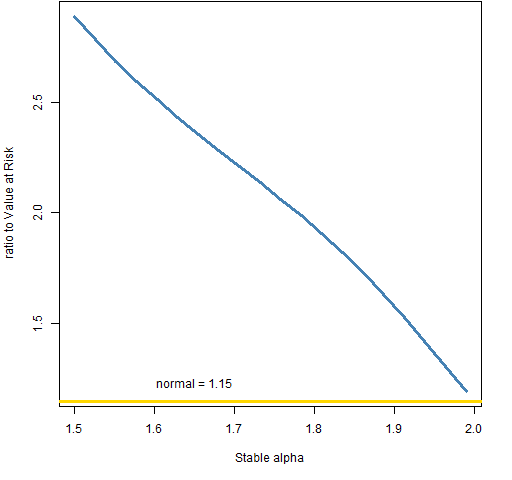# The scaling of Expected Shortfall

June 16, 2013
By

Want to share your content on R-bloggers? click here if you have a blog, or here if you don't.

Getting Expected Shortfall given the standard deviation or Value at Risk.

## Previously

There have been a few posts about Value at Risk and Expected Shortfall.

Properties of the stable distribution were discussed.

## Scaling

One way of thinking of Expected Shortfall is that it is just some number times the standard deviation, or some other number times the Value at Risk.  Those numbers, of course, depend on the distribution that is being assumed.

### t distribution

Figures 1 through 4 show scalings for t distributions with degrees of freedom from 3 to 20.

Figure 1: 95% Expected Shortfall relative to the standard deviation for t-distributions.The value for 3 degrees of freedom is almost  the same as for 5 degrees of freedom.

Figure 2: 99% Expected Shortfall relative to the standard deviation for t-distributions.Figure 3: 95% Expected Shortfall relative to Value at Risk for t-distributions.Figure 4: 99% Expected Shortfall relative to Value at Risk for t-distributions.### stable distribution

Figures 5 and 6 show ratios to the Value at Risk for values of the stable distribution alpha that are equally spaced between 1.5 and 1.99.  The standard deviation is infinite for these distributions.

Figure 5: 95% Expected Shortfall relative to Value at Risk for stable distributions.Figure 6: 99% Expected Shortfall relative to Value at Risk for stable distributions.## Summary

The range of values in these plots tells us that we are unlikely to know more than one significant digit of the Expected Shortfall even if we knew the Value at Risk exactly (which we don’t — by a long shot).

The true Expected Shortfall is probably not more than three times the Value at Risk.

## Epilogue

‘ Cause the dawn and the dying light
Have gathered too much meaning

from “Long Ride” by Taasha Coates and Tristan Goodall

## Appendix R

Computations were done in R.

#### normal distribution

We just need to do an integration and remember to divide by the probability of being in the interval.

```sscaleESnorm05 <- -integrate(function(x)
x * dnorm(x), -Inf, qnorm(.05))\$value /
.05```

This is the ratio to the standard deviation (which is 1 in this case).

#### t distribution

The function to compute the ratios to the standard deviation is:

```pp.tscaleES <- function(df, level)
{
# placed in the public domain 2013
# by Burns Statistics

# get scaling of ES relative to
# standard deviation

# testing status: very mildly tested

level <- ifelse(level > .5, 1 - level,
level)
ans <- array(NA,
c(length(df), length(level)),
list(df, level))
for(i in seq(along=df)) {
for(j in seq(along=level)) {
tdf <- df[i]; tlev <- level[j]
ans[i,j] <- -integrate(function(x)
x * dt(x, tdf) * sqrt((tdf - 2)/tdf),
-Inf, qt(tlev, tdf))\$value / tlev
}
}
ans
}```

This is used like:

`sscaletES <- pp.tscaleES(3:20, c(.05, .01))`

#### stable distribution

The function for the stable distribution is modeled on the one for the t.  However, there is no standard deviation so the ratio to the Value at Risk is computed.

```pp.stablescaleES <- function(alpha, level)
{
# placed in the public domain 2013
# by Burns Statistics

# get scaling of ES relative to VaR

# testing status: very mildly tested

require(stabledist)

level <- ifelse(level > .5, 1 - level,
level)
ans <- array(NA,
c(length(alpha), length(level)),
list(alpha, level))
for(i in seq(along=alpha)) {
for(j in seq(along=level)) {
talpha <- alpha[i]; tlev <- level[j]
tnvar <- qstable(tlev, alpha=talpha,
beta=0)
ans[i,j] <- -integrate(function(x)
x * dstable(x, alpha=talpha, beta=0),
-Inf, tnvar)\$value / tlev / -tnvar
}
}
ans
}```

The function was used like:

```vscalestabES <- pp.stablescaleES(seq(1.5,
1.99, length=20), c(.05, .01))```R-bloggers.com offers daily e-mail updates about R news and tutorials about learning R and many other topics. Click here if you're looking to post or find an R/data-science job.
Want to share your content on R-bloggers? click here if you have a blog, or here if you don't.